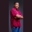Related Tags

swift
octal
communitycreator

# How to create a binary and print the value in decimal in SwiftTheodore Kelechukwu Onyejiaku

### Overview

We can create a constant in Swift using the let keyword. It is also easy to create an octal binary number, that is a number in base 2. All we need to achieve this is to place the 0b before the binary digits.

### Syntax

let constant = 0b(binary_digit)
The syntax for creating a constant with binary as value

### Parameters

• let: This is the keyword used in creating a constant in swift.
• constant: This is the name of the variable we want to create as a constant.
• 0b : This is the keyword used to express a binary value.
• binary_digit : This represents the binary digit or digits.

### Return value

The value returned is a binary value.

### Example

// create some binary values
let binary1 = 0b1
let binary2 = 0b11101
let binary3 = 0b101010
let binary4 = 0b111111

// print binary values as decimals
print(binary1);  // 1
print(binary2);  // 29
print(binary3);  // 42
print(binary4);  // 63
The Swift program to print a binary in decimal

### Explanation

• Lines 2-5: We create some constant binary variables and assign them binary values using the 0b.
• Lines 8-11: We print the binary values, in the form of decimals, to the console.

RELATED TAGS

swift
octal
communitycreator

CONTRIBUTORTheodore Kelechukwu Onyejiaku
RELATED COURSES

View all Courses

Keep Exploring

Learn in-demand tech skills in half the time